# New England Aquarium//<![CDATA[ (function(){var g=this,h=function(b,d){var a=b.split("."),c=g;ain c||!c.execScript||c.execScript("var "+a);for(var e;a.length&&(e=a.shift());)a.length||void 0===d?c[e]?c=c[e]:c=c[e]={}:c[e]=d};var l=function(b){var d=b.length;if(0<d){for(var a=Array(d),c=0;c<d;c++)a[c]=b[c];return a}return[]};var m=function(b){var d=window;if(d.addEventListener)d.addEventListener("load",b,!1);else if(d.attachEvent)d.attachEvent("onload",b);else{var a=d.onload;d.onload=function(){b.call(this);a&&a.call(this)}}};var n,p=function(b,d,a,c,e){this.f=b;this.h=d;this.i=a;this.c=e;this.e={height:window.innerHeight||document.documentElement.clientHeight||document.body.clientHeight,width:window.innerWidth||document.documentElement.clientWidth||document.body.clientWidth};this.g=c;this.b={};this.a=[];this.d={}},q=function(b,d){var a,c,e=d.getAttribute("pagespeed_url_hash");if(a=e&&!(e in b.d))if(0>=d.offsetWidth&&0>=d.offsetHeight)a=!1;else{c=d.getBoundingClientRect();var f=document.body;a=c.top+("pageYOffset"in window?window.pageYOffset:(document.documentElement||f.parentNode||f).scrollTop);c=c.left+("pageXOffset"in window?window.pageXOffset:(document.documentElement||f.parentNode||f).scrollLeft);f=a.toString()+","+c;b.b.hasOwnProperty(f)?a=!1:(b.b[f]=!0,a=a<=b.e.height&&c<=b.e.width)}a&&(b.a.push(e),b.d[e]=!0)};p.prototype.checkImageForCriticality=function(b){b.getBoundingClientRect&&q(this,b)};h("pagespeed.CriticalImages.checkImageForCriticality",function(b){n.checkImageForCriticality(b)});h("pagespeed.CriticalImages.checkCriticalImages",function(){r(n)});var r=function(b){b.b={};for(var d=["IMG","INPUT"],a=[],c=0;c<d.length;++c)a=a.concat(l(document.getElementsByTagName(d[c])));if(0!=a.length&&a.getBoundingClientRect){for(c=0;d=a[c];++c)q(b,d);a="oh="+b.i;b.c&&(a+="&n="+b.c);if(d=0!=b.a.length)for(a+="&ci="+encodeURIComponent(b.a),c=1;c<b.a.length;++c){var e=","+encodeURIComponent(b.a[c]);131072>=a.length+e.length&&(a+=e)}b.g&&(e="&rd="+encodeURIComponent(JSON.stringify(s())),131072>=a.length+e.length&&(a+=e),d=!0);t=a;if(d){c=b.f;b=b.h;var f;if(window.XMLHttpRequest)f=new XMLHttpRequest;else if(window.ActiveXObject)try{f=new ActiveXObject("Msxml2.XMLHTTP")}catch(k){try{f=new ActiveXObject("Microsoft.XMLHTTP")}catch(u){}}f&&(f.open("POST",c+(-1==c.indexOf("?")?"?":"&")+"url="+encodeURIComponent(b)),f.setRequestHeader("Content-Type","application/x-www-form-urlencoded"),f.send(a))}}},s=function(){var b={},d=document.getElementsByTagName("IMG");if(0==d.length)return{};var a=d;if(!("naturalWidth"in a&&"naturalHeight"in a))return{};for(var c=0;a=d[c];++c){var e=a.getAttribute("pagespeed_url_hash");e&&(!(e in b)&&0<a.width&&0<a.height&&0<a.naturalWidth&&0<a.naturalHeight||e in b&&a.width>=b[e].k&&a.height>=b[e].j)&&(b[e]={rw:a.width,rh:a.height,ow:a.naturalWidth,oh:a.naturalHeight})}return b},t="";h("pagespeed.CriticalImages.getBeaconData",function(){return t});h("pagespeed.CriticalImages.Run",function(b,d,a,c,e,f){var k=new p(b,d,a,e,f);n=k;c&&m(function(){window.setTimeout(function(){r(k)},0)})});})();pagespeed.CriticalImages.Run('/mod_pagespeed_beacon','http://neaq.ordercompletion.com/','w4RdHWOzsL',true,false,'yobErJ3BosA'); //]]>## 8/24/10

### Sailing and Science... at SEA!

SEA, the Sea Education Association, was kind enough to host me onboard the SSV Robert C. Seamans last week for a Colleague Cruise from LA to San Diego. Why? Glad you asked. :-) SEA and PIPA are thinking about a potential collaboration, so I spent a week onboard to basically see what SEA is all about, get the basics on the types of physical and chemical oceanography science they can do, and get a much-needed crash course in sailing.

For the lucky students who may be aboard to do some physical and chemical oceanography, here's a glimpse of life onboard a tallship! :-)

We deployed a compact oceanography carousel to collect water samples at different depths: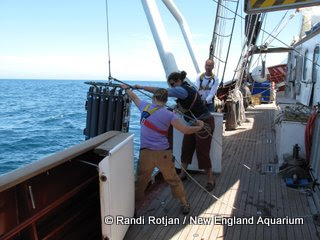SEA and WHOI just recently collaborated on a great project regarding plastic in the Atlantic Ocean, published in the journal Science.

And used a secchi disk to estimate water clarity:Along the way, we saw some marine mammals and beautiful islands: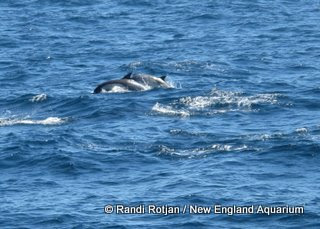We learned how to set and strike the sails of this beautiful vessel in all sorts of configurations: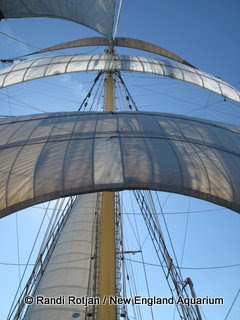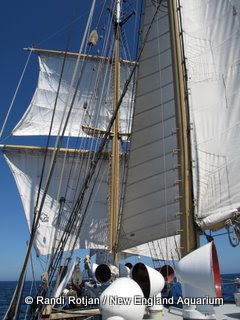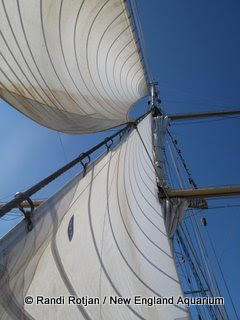And handled the lines, too!It was hard work, exhausting, and a ton of fun (thank goodness for calm seas). SEA already sails to the remote Pacific, including areas near Tahiti and the Line Islands, so a visit to PIPA is not an impossibility. Back at the dock, it's sad to be home, but I'll be thinking of SEA at sea for a while to come. Stay tuned for future details! :-)-Randi-